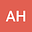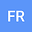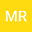A modification on the exponential cubic B-spline for numerical simulation of hyperbolic telegraph equations
•••Forouzan Rahimian
Urmia University of Technology
Author ProfileMajid Roohi
Guangzhou University
Author Profile## Abstract

In this paper, the differential quadrature method is implemented to find the numerical solution of two and three-dimensional telegraphic equations‏ with Dirichlet and Neumann’s boundary values. This technique is according to exponential cubic B-spline functions. So, a modification on the exponential cubic B- spline is applied in order to use as a basis function in the DQ method. Therefore, the Telegraph equation (TE) is altered to a system of ordinary differential equations (ODEs). The optimized form of Runge-Kutta scheme has been implemented by four-stage and three-order strong stability preserving (SSP-RK43) to solve the resulting system of ODEs. We examined the correctness and applicability of this method by four examples of the TE.